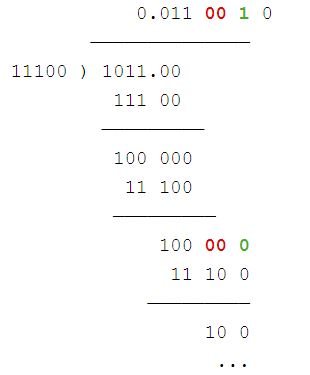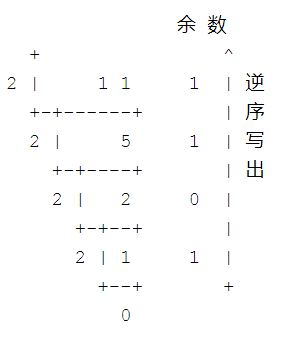• 此示例后面的示例演示了如何将十进制转换为二进制值。 十六进制数字是一个字符串，其中包含介于0到9之间的数字和字符AF； 其中A = 10，B = 11，...，F =15。（有关更多信息，请查询（google）十六进...

二进制十六进制十进制转换 将十六进制数转换为十进制数，并将十进制数转换为十六进制数  这是一个非常简单的脚本，可将十进制数字转换为十六进制值，并将十六进制值转换为十进制数字。 此示例后面的示例演示了如何将十进制数转换为二进制值。
十六进制数字是一个字符串，其中包含介于0到9之间的数字和字符AF； 其中A = 10，B = 11，...，F =15。（有关更多信息，请查询（google）十六进制数字。）
考虑到这一点，很明显，您需要调用Number对象的  toString（）方法 ，以便将Number转换为十六进制值。 当您调用toString（）方法时，只需将其作为参数传递16，它将以基数16（十六进制）显示数字。  要将十六进制数字转换为十进制数字，可以使用  parseInt（）方法 。 您通过此方法将包含十六进制值的字符串作为第一个参数，将16作为第二个参数，以指示该字符串是一个以16为底的数字。 这将返回一个数字对象，该对象是等于十六进制值的十进制（以10为底）。 
<html xmlns="http://www.w3.org/1999/xhtml">
<head></head>
<body>
<script type="text/javascript">
function ConvertToHex(){
var numberValue = document.getElementById('NumberInput').value;
var decNumber = Number(numberValue);
var hexNumber = decNumber.toString(16).toUpperCase();
document.getElementById('Result').value = hexNumber;
}
function ConvertToDec(){
var hexNumber = document.getElementById('NumberInput').value;
var decNumber = parseInt(hexNumber,16);
document.getElementById('Result').value = decNumber;
}
</script>
<div style="text-align:center">
Number: <input type="text" id="NumberInput"></input>
Result: <input type="text" id="Result"></input>
<br/>
<button onclick="ConvertToHex();">Convert To Hex</button>
<button onclick="ConvertToDec();">Convert To Decimal</button>
<br />
</div>
</body>
</html>将二进制数转换为十进制数，并将十进制数转换为二进制数  此技术可用于将数字转换为所需的任意基数！ 例如，如果要将十进制数转换为二进制，则只需使用2而不是16：

<html xmlns="http://www.w3.org/1999/xhtml">
<head></head>
<body>
<script type="text/javascript">
function ConvertToBinary(){
var numberValue = document.getElementById('NumberInput').value;
var decNumber = Number(numberValue);
var binaryNumber = decNumber.toString(2).toUpperCase();
document.getElementById('Result').value = binaryNumber;
}
function ConvertToDec(){
var binaryNumber = document.getElementById('NumberInput').value;
var decNumber = parseInt(binaryNumber, 2);
document.getElementById('Result').value = decNumber;
}
</script>
<div style="text-align:center">
Number: <input type="text" id="NumberInput"></input>
Result: <input type="text" id="Result"></input>
<br/>
<button onclick="ConvertToBinary();">Convert To Binary</button>
<button onclick="ConvertToDec();">Convert To Decimal</button>
<br />
</div>
</body>
</html>编码愉快！  -弗林尼
翻译自: https://bytes.com/topic/javascript/insights/867841-converting-hex-binary-decimal-decimal-hex-binary-javascript-example二进制十六进制十进制转换

展开全文字符串 javascript java python js
• 此示例后面的示例演示了如何将十进制转换为二进制值。 十六进制数字是一个字符串，其中包含介于0到9之间的数字和字符AF； 其中A = 10，B = 11，...，F =15。（有关更多信息，请查询（google）十六进制数字。） ...
 将十六进制数转换为十进制数，并将十进制数转换为十六进制数  这是一个非常简单的脚本，可将十进制数字转换为十六进制值，并将十六进制值转换为十进制数字。 此示例后面的示例演示了如何将十进制数转换为二进制值。  十六进制数字是一个字符串，其中包含介于0到9之间的数字和字符AF； 其中A = 10，B = 11，...，F =15。（有关更多信息，请查询（google）十六进制数字。）  考虑到这一点，很明显，您需要调用Number对象的toString（）方法才能将Number转换为十六进制值。 当您调用toString（）方法时，只需将其作为参数传递16，它将以基数16（十六进制）显示数字。  要将十六进制数转换为十进制数，可以使用parseInt（）方法 。 您通过此方法将包含十六进制值的字符串作为第一个参数，将16用作第二个参数，以指示该字符串是一个以16为底的数字。 这将返回一个数字对象，该对象是与十六进制值等效的十进制（以10为底）。 
<html xmlns="http://www.w3.org/1999/xhtml">
<head></head>
<body>
<script type="text/javascript">
function ConvertToHex(){
var numberValue = document.getElementById('NumberInput').value;
var decNumber = Number(numberValue);
var hexNumber = decNumber.toString(16).toUpperCase();
document.getElementById('Result').value = hexNumber;
}
function ConvertToDec(){
var hexNumber = document.getElementById('NumberInput').value;
var decNumber = parseInt(hexNumber,16);
document.getElementById('Result').value = decNumber;
}
</script>
<div style="text-align:center">
Number: <input type="text" id="NumberInput"></input>
Result: <input type="text" id="Result"></input>
<br/>
<button onclick="ConvertToHex();">Convert To Hex</button>
<button onclick="ConvertToDec();">Convert To Decimal</button>
<br />
</div>
</body>
</html>将二进制数转换为十进制数，并将十进制数转换为二进制数  此技术可用于将数字转换为所需的任意基数！ 例如，如果要将十进制数转换为二进制，则只需使用2而不是16： 
<html xmlns="http://www.w3.org/1999/xhtml">
<head></head>
<body>
<script type="text/javascript">
function ConvertToBinary(){
var numberValue = document.getElementById('NumberInput').value;
var decNumber = Number(numberValue);
var binaryNumber = decNumber.toString(2).toUpperCase();
document.getElementById('Result').value = binaryNumber;
}
function ConvertToDec(){
var binaryNumber = document.getElementById('NumberInput').value;
var decNumber = parseInt(binaryNumber, 2);
document.getElementById('Result').value = decNumber;
}
</script>
<div style="text-align:center">
Number: <input type="text" id="NumberInput"></input>
Result: <input type="text" id="Result"></input>
<br/>
<button onclick="ConvertToBinary();">Convert To Binary</button>
<button onclick="ConvertToDec();">Convert To Decimal</button>
<br />
</div>
</body>
</html>编码愉快！  -弗林尼 From: https://bytes.com/topic/javascript/insights/867841-converting-hex-binary-decimal-decimal-hex-binary-javascript-example

展开全文• ﻿﻿ 整数和小数分别转换。 整数除以2，商继续除以2，得到0为止，将余数逆序排列。...所以22的二进制是10110 小数乘以2，取整，小数部分继续乘以2，取整，得到小数部分0为止，将整数顺序排列。 0.8125x2=
﻿﻿
整数和小数分别转换。
整数除以2，商继续除以2，得到0为止，将余数逆序排列。
22 / 2  11 余0
11/2     5  余 1
5 /2      2  余 1
2 /2      1  余 0
1 /2      0  余 1
所以22的二进制是10110

小数乘以2，取整，小数部分继续乘以2，取整，得到小数部分0为止，将整数顺序排列。
0.8125x2=1.625 取整1,小数部分是0.625
0.625x2=1.25 取整1,小数部分是0.25
0.25x2=0.5 取整0,小数部分是0.5
0.5x2=1.0 取整1,小数部分是0，结束
所以0.8125的二进制是0.1101
十进制22.8125等于二进制10110.1101

展开全文• ## 十进制小数分数与二进制的转换

万次阅读 多人点赞 2017-01-13 19:37:26
例如将十进制分数11/28转换为二进制数，过程如下： 1、首先将分子分母分别转换成二进制 （11）10=（1011）2 （28）10=（11100）2 2、使用短除，借位时是借2，商只能是0或1 所以：11/28=1011/11100=0.01100100.....


十进制分数转换为二进制数
使用短除法。
例如将十进制分数11/28转换为二进制数，过程如下：
1、首先将分子分母分别转换成二进制
（11）10=（1011）2
（28）10=（11100）2

2、使用短除，借位时是借2，商只能是0或1所以：11/28=1011/11100=0.01100100...十进制小数转换为二进制小数
十进制数的整数位是二进制数的整数位，十进制数的小数位是二进制数的小数位。两部分分开转换。
整数部分 除以2取余，逆序排列。
小数部分 乘 2 取整，顺序排列。
例如转换十进制小数11.4，过程如下。
计算整数部分，11转换为二进制位1011：计算小数部分0.4，首先将小数部分一直乘2，积的整数部分顺序取出：
0.4*2=0.8      取0      |
0.8*2=1.6      取1      |  顺
0.6*2=1.2      取1      |  序
0.2*2=0.4      取0      |  排
0.4*2=0.8      取0      |  列
0.8*2=1.6      取1      |
0.6*2=1.2      取1      |
0.2*2=0.4      取0      |
可以看出0110是循环，因此小数部分的二进制是
0.01100110……（循环0110）
最终结果是整数位和小数位合并1101111.01100110……（2）

二进制小数转换为十进制小数
使用按权展开求和法，小数点左边是2的正数次方，从0开始；小数点右边是2的负数次方，从-1开始。
例如将101.111(2)转换成十进制数
1*(2^2)+0*(2^1)+1*(2^0)       # 整数部分

+ 1*(2^(-1))+1*(2^(-2))+1*(2^(-3))       # 小数部分

=5.875

附 python 版十进制与二进制转换

# coding=UTF-8
from decimal import Decimal

def dec2bin(n, bit=20):
"""
n, integer or float to convert
bit, bits after point
return binary, string
"""
negative = False
if n < 0:
negative = True
n *= -1

integer = int(n)
decimal = n - integer
binary = ""

if n == 0:
return "0"

while integer != 0:
result = int(integer % 2)
integer /= 2
binary = str(result) + binary

if decimal != 0:
i = 0
decimal_bin = ""  # binary decimal after convert
while decimal != 0 and i < bit:
result = int(decimal * 2)
decimal = decimal * 2 - result
decimal_bin += str(result)
i += 1
binary = binary + '.' + decimal_bin

if negative:
binary = '-' + binary

return binary

def bin2dec(n):
"""
n binary, support point
return integer or float
"""
negative = False
if n < 0:
negative = True
n *= -1

integer = int(n)
decimal = n - integer

if integer != 0:
integer_str = str(integer)
length = len(integer_str)

integer = 0
for i in xrange(0, length):
bit = int(integer_str[i])
if bit == 1:
integer += 2 ** (length - i - 1)
elif bit != 0:
print "invalid integer:" + str(n)

if decimal != 0:
decimal_str = str(decimal)[2:] # skip "0."
length = len(decimal_str)

decimal = 0
for i in xrange(0, length):
bit = int(decimal_str[i])
if bit == 1:
decimal += 2 ** (-1 * (i + 1))
elif bit != 0:
print "invalid decimal:" + str(n)

result = integer + decimal

if negative:
result *= -1

return result

def testcases():
for pair in [(125, '1111101'),
(1.3, '1.01001100110011001100'),
(2.5, '10.1'),
(0, '0'),
(-1, '-1'),
(0.5, '.1')]:
assert pair == dec2bin(pair)

for pair in [(-1001.1100, -9.75),
(-1101, -13),
(111.111, 7.875),
(0.1101, 0.8125),
(1001, 9),
(0, 0),
(0.1, 0.5)]:
assert pair == bin2dec(pair)

print "all test case success"

if __name__ == "__main__":
# testcases()
print bin2dec(0.5)


参考：

http://blog.csdn.net/caoguo_app_android/article/details/9955743


展开全文• 十进制转换为二进制和十六进制 一.十进制数转化为二进制 整数除以2，商继续除以2，得到0为止。 以23为例： 23/2 11余1 11/2 5 余 1 5 /2 2余 1 2 /2 1余 0 1 /2 0余 1 对于其余数从上到下为二进制的低位到高位;...
• 我们可以使用Integer类的toString（）方法将十进制转换为二进制数。此方法有两个参数，第一个参数是十进制数，第二个参数是我们要转换的基数。对于二进制，基数为2。 1 2 3 4 5 ...
• 从基本十进制转换为二进制基 转换步骤： 将数字除以2。 获取下一次迭代的整数商。 获取其余的二进制数字。 重复这些步骤，直到商等于0。 十进制到二进制的转换示例。 (51)10 = (110011)2. (217)10 = ...D
• 输入一个十进制数N，把它转换成R进制数输出。 【Input】输入数据包含多个测试实例，每个测试实例包含两个整数N（int范围）和R（2<=R<=16，R<>10）。 【Ouput】每个测试实例输出转换后的数，每个输出占...算法 聚类算法
• JAVA中的十进制转换为二进制关注:106答案:5mip版解决时间 2021-02-08 10:44提问者这笑，有多危险2021-02-07 11:41不要用函数最佳答案二级知识专家此生不换的執著2021-02-07 12:59public class test {public static ...
• 二、十进制转换为二进制十进制转换为二进制数时，由于整数和小数的转换方法不同，所以先将十进制数的整数部分和小数部分分别转换后，再加以合并。1. 十进制整数转换为二进制整数十进制整数转换为二进制整数采....
• 一、十进制整数转换为二进制 一般方法：对所给数字除以2进行求余，然后从最后一个余数读到第一个余数，以11为例。 可求得其二进制为：1011 针对不太大的数字，我们可以用简便方法。 首先，我们解析一下十进制数字，...
• 二、十进制转换为二进制十进制转换为二进制数时，由于整数和小数的转换方法不同，所以先将十进制数的整数部分和小数部分分别转换后，再加以合并。1. 十进制整数转换为二进制整数十进制整数转换为二进制整数采....
• 十进制转换为二进制数字为例子 十进制 二进制 1 1 2 10 3 1%1 10 1010 二.以十进制位获得每一位数字为例子 1 -----> 1%10=1 11 -----> 11%10=1 + (11/10 )%10=1 123-----> 123%10=3 + ( ...
• 文章目录定义一、二进制数转换成十进制数二、十进制转换为二进制数1. 十进制整数转换为二进制整数2．十进制小数转换为二进制小数 定义 进制也就是进位计数制，是人为定义的带进位的计数方法。 十进制是逢十进一，...java
• （Tip：十进制小数转换二进制用乘2取整）解题思路：分为整数部分转换和小数部分转换，两步操作整数部分除2逆向取余小数部分乘2正向取整如：12.45=1100.0.011100110012/2=6..06/2=3..03/2=1..11/2=0..1结果11000....c语言
• 十进制整数转换二进制数 对于每个n，以11位的宽度右对齐输出n值，然后输出"-->"，然后输出二进制数。 输入样例： 2 0 -12 1 输出样例：  2-->10  0-->0  -12-->-1100  1-->1 #include #...C++
• 转：十进制小数转化为二进制小数 一、二进制数转换成十进制数  由二进制数转换成十进制数的基本做法是，把二进制数首先... 十进制转换为二进制数时，由于整数和小数的转换方法不同，所以先将十进制数的整数部分和
• 科学计数法 一、二进制数转换成十进制数  由二进制数转换成十进制数的基本做法是，把二进制数首先... 十进制转换为二进制数时，由于整数和小数的转换方法不同，所以先将十进制数的整数部分和小数部分分别转换c++ c语言
• 1将十进制转换为二进制 #include<stdio.h> /* *csdn学院--2016级 *目的：让代码见证成长(作为一个初学的菜鸟，如 *大家有发现错误，欢迎指正！) *文件名称:Myfun203.c *问题描述:用C语言将十进制转换为二...
• 二、十进制转换为二进制十进制转换为二进制数时，由于整数和小数的转换方法不同，所以先将十进制数的整数部分和小数部分分别转换后，再加以合并。1. 十进制整数转换为二进制整数十进制整数转换为二进制整数采....
• 十六进制的转换为十进制两种常见方法 好久以前学的都忘了，正好复习一下 ...将十六进制转换为二进制在转换为十进制（第一种小伙伴们或许不好理解，第二种容易理解） 比如： 1A首先转为二进制：0001 1010 0算法
• 1.正整数的十进制转换为二进制.  十进制整数转换为二进制整数采用"除2取余，逆序排列"法。具体做法是：用2整除十进制整数，可以得到一个商和余数；再用2去除商，又会得到一个商和余数，如此进行，直到商为0时为止，...基础知识
• 今天没事儿刷了个题，本以为是十分easy的题目，结果结实把我恶心了一阵。...a = 11b = 1返回 100解法：很简单，先把二进制转换为十进制，然后加和，然后再转换为2进制。需要注意的是，二进制to十进制很容
• 进制也就是进制位，对于接触过电脑的人来说应该都不陌生，我们常用的进制...常用进制介绍如下：二进制 二进制，逢二进一，数字中只有 0 和 1例如，数数，二进制的数法是：0，1 接着 10，11 接着 100，101，110，111 ...
• 二进制数据是用0和1两个数码来表示的数。它的基数为2，进位规则是“逢二进一”，借位规则是“借一当二”，如：8位二进制数：...c语言中十六进制数前加“0x”表示，例如：十六进制：0x35 转换为二进制数为：00110101...